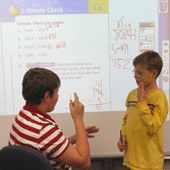Standards of Mathematical Practice

3. Construct viable arguments and critique the reasoning of others.Mathematically proficient students understand and use stated assumptions, definitions, and previously established results in constructing arguments.
I can...
 CONSTRUCT arguments using concrete objects, drawings, diagrams, and actions. LISTEN to the arguments of others. QUESTION to clarify or improve the arguments. READ the arguments of others. JUSTIFY my answers. RESPOND to the arguments of others. CRITIQUE the arguments of others.

4 . Model with mathematics.Mathematically proficient students can apply the mathematics they know to solve problems arising in everyday life, society, and the workplace.
I can...
 WRITE equations to describe a situation. DRAW pictures, models, and/or graphic representations. IDENTIFY important quantities in a problem. REPRESENT the problem using manipulatives and/or equations. INTERPRET my mathematical results in context. EVALUATE my solution(s) and make changes if needed.